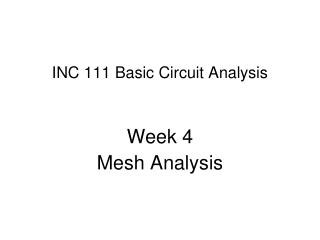DownloadDownload PresentationINC 111 Basic Circuit Analysis

# INC 111 Basic Circuit Analysis

Télécharger la présentation## INC 111 Basic Circuit Analysis

- - - - - - - - - - - - - - - - - - - - - - - - - - - E N D - - - - - - - - - - - - - - - - - - - - - - - - - - -
##### Presentation Transcript

1. INC 111 Basic Circuit Analysis Week 4 Mesh Analysis

2. Mesh Analysis (Loop Analysis) Mesh = A closed loop path which has no smaller loops inside

3. Mesh Analysis • Procedure • Count the number of meshes. Let the number equal N. • Define mesh current on each mesh. Let the values beI1, I2, I3, … • Use Kirchoff’s voltage law (KVL) on each mesh, generating N equations • Solve the equations

4. I2 I1 Mesh current (loop current) Example Use mesh analysis to find thepower consumption inthe resistor 3 Ω

5. I2 I1 Loop 1 Equation 1 Loop 2 Equation 2 I1 = 6A, I2 = 4A, The current that pass throughR 3Ω is6-4 = 2A (downward) Power = 12 W

6. I2 I1 I3 Example Use Mesh analysis to findVx

7. I2 Equation 1 I1 I3 Equation 2 Equation 3 I1 = 3A, I2 = 2A, I3 = 3A Vx = 3(I3-I2) = 3V

8. Supermesh When there is a current source in the mesh path, we cannot use KVL because we do not know the voltage across the current source. We have to use supermesh, which is a combination of 2 meshes to be a big mesh, and avoid the inclusion of the current source in the mesh path.

9. I2 I1 I3 Example UseMesh analysis to findVx

10. I2 I1 I3 Equation from 2nd loop

11. Supermesh I2 I1 I3 Equation 2 Equation 3

12. I1 = 9A I2 = 2.5A I3 = 2A Vx = 3(I3-I2) = -1.5V

13. How to choose betweenNode and Mesh Analysis The hardest part in analyzing circuits is solving equations. Solving 3 or more equations can be time consuming. Normally, we will count the number of equations according to each method and select the method that have lesser equations.

14. Example From the previous example, if we want to use Nodal Analysis 7V V2 V1 V3 0V

15. I2 I1 I3 Example: Dependent Source Find Vx

16. I2 I1 I3 Equation 1 Equation 2 Equation 3 Equation 4 I1=15A, I2=11A, I3=17A Vx = 3(17-11) = 18V

17. Special Techniques • Superposition Theorem • Thevenin’s Theorem • Norton’s Theorem • Source Transformation

18. Superposition Theorem In a linear circuit, we can calculate the value of current (or voltage) that is the result from each voltage source and current source independently. Then, the real value is the sum of all current (or voltage) from the sources.

19. Linearity V and I have linear relationship I V

20. Implementation • When calculating the effect of a source, the other sources will be set to zero. • For voltage sources, when set as 0V, it will be similar to short circuit • For current sources, when set as 0A, it will be similar to open circuit

21. Example I1 = 1A I2 = 2A I total = 1+2 = 3A

22. Example I1 = 1A I2 = 0A I total = 1+0 = 1A

23. Example Find voltage Vx

24. Superposition andDependent Source Dependent sources cannot be used with superposition. They have to be active all the time.

25. Example Usesuperposition to findIx

26. KVL FindIx byeliminating the current source 3A

27. Ix+3 KVL outer loop FindIx by eliminating the voltage source10V